Study S3 A Math Quadratic Functions in Real-World Context - Geniebook# Quadratic Functions In Real-World Context

• conditions for the Quadratic curve to lie completely above or below the x−axis.
• modelling real−world situations using quadratic functions.

## Completing The Square

Question 1:

Express the equation $$2x^2 -8x +10$$ in the form $$a(x-h)^2+k$$.

Solution:

We will need to factorise the coefficient of $$x^2$$ before we can complete the square.

$$2x^2-8x+10=2[x^2-4x+5]$$

To complete the square, we need to express in the form of $$a^2-2ab+b^2$$. To do so, we will half the value of the coefficient of $$x$$ to obtain the value of $$b$$. To maintain the equality of the expression, we will add another $$−2^2$$ term to neutralise the term that we have added.

\begin{align} &2[x^2-4x\; \overbrace{+\;2^2-2^2\;+}^0\;5]\\ &\quad^\underbrace{\qquad\qquad\quad}\\ &= 2[(x-2)^2-2^2+5]\\ &= 2[(x-2)^2+1]\\ &=2(x-2)^2+2 \end{align}

## Sketching Graphs Of Quadratic Function

Sketching Quadratic Functions in the form $$a(x-h)^2+k$$.

When we sketch a Quadratic graph for the function $$a(x-h)^2+k$$ with the value of $$a<0$$  then we have a downward open parabola. You can remember it as the n−shaped graph that has a maximum point. Below is an example of a quadratic graph with $$a<0$$ with $$h=0,k=0$$.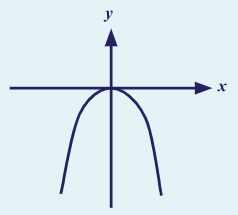However, when we sketch the same graph for a positive value of $$a$$, where $$a=3$$, in that case, the graph obtained is an upward open parabola. You can remember it as a u−shaped graph that has a minimum point. Below is an example of a quadratic graph with $$a>0$$ with $$h=0,k=0$$.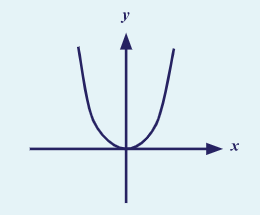Let’s consider the u−shaped graph given above. When we change the value of $$h$$, we see there is a change in the position of the graph along the x−axis. When the value of $$h$$ is positive the graph shifts towards the positive x−axis or towards the right of the graph.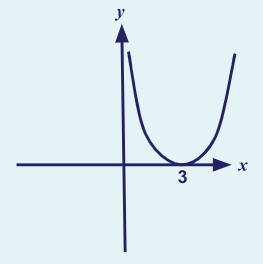When the value of $$h$$ is negative, it shifts towards the negative x−axis which is towards the left of the graph.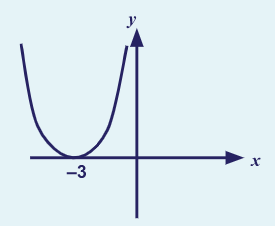However, when the value of $$k$$ changes it makes the graph shift along the y−axis.

A positive value of $$k$$ makes the graph shift along the positive y−axis or upwards and a negative value of $$k$$ shifts the graph towards the negative y−axis or in a downwards direction.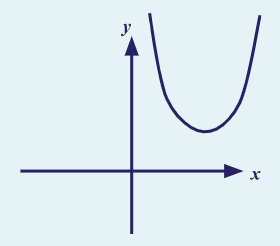You can summarise the entire point using this image: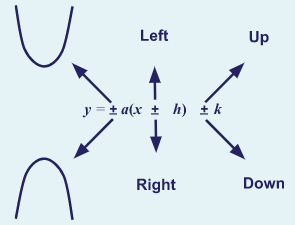Question 2:

Sketch the graph of $$y=2(x-1)^2-3$$, indicating clearly the coordinates of the turning point and the values where the graph crosses the x & y−axes.

Solution:

Let us look at information that has been provided to us.

$$y=2(x-1)^2-3$$

Upon looking at the equation, we get the value of $$a=2, h=1$$ & $$k=-3$$.

Looking at the value of $$a$$, we understand immediately that our graph will be an upward open parabola graph.

The values of $$h$$ and $$k$$ show that the graph will shift by 1 point towards the right along the positive $$x$$−axis and downwards by 3 points along negative $$y$$ direction. Hence, the minimum point of the graph will naturally be at (1,−3).

To find the $$y$$−intercept for this we can simply take the equation and substitute $$x=0$$.

\begin{align}​​ y &=2(x-1)^2-3\\ \implies y &=2(0-1)^2-3\\ \implies y &=2-3\\ \implies y &=-1 \end{align}

Therefore, the y−intercept is  $$−1$$.

Similarly for x−intercept substituting $$y=0$$ results in

\begin{align}​​ y &=2(x-1)^2-3\\ \implies \qquad\quad 0 &=2(x-1)^2-3\\ \implies \qquad\quad 3 &=2(x-1)^2\\ \implies \qquad\; 1.5 &=(x-1)^2 \\ \implies (x-1)^2 &=1.5\\ \implies \;\quad x-1 &=\sqrt{1.5}\\ \implies \qquad\quad x &= 1 \pm 1.22\\ \implies \qquad \quad x &= 2.22 \qquad\text{or}\qquad -0.22 \end{align}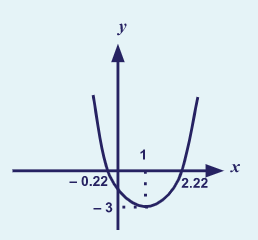Example 1:

Without sketching the graph, explain whether $$y = -2x^2+4x -5$$ lies completely above or below the x−axis.

Solution:

First, we need to convert the equation into the square form by completing the square.

\begin{align}​​ y &= -2x^2+4x -5\\ \implies y &= -2[x^2-2x +2.5]\\ \implies y &= -2[x^2-2x+1^2-1^2 +2.5]\\ \implies y &= -2[(x-1)^2-1^2 +2.5] & \text{​(upon dividing coefficient of x by 2)​}\\ \implies y &= -2[(x-1)^2+1.5]\\ \implies y &= -2(x-1)^2-3\\ \end{align}

• Now that we have obtained the square form, we see the shape of the graph as n−shaped since the value of $$a=-2$$, which is negative.
• Then we find out the maximum point coordinates by looking at the values of $$h$$ and $$k$$ obtained when we compare the obtained equation with the standard equation.
• The value of $$h=1$$ and hence the graph shifts right along $$x$$−axis by 1 point.
• The value of $$k=-3$$ and hence the graph shifts down by 3 points.

Since the graph of $$y = -2x^2+4x -5$$ has maximum point at (1, 3), hence the curve lies completely below the $$x$$−axis.

Question 3:

Without sketching the graph, explain whether $$y=3x^2 +12x +17$$ lies completely above or below the x−axis.

Express $$y=3x^2 +12x +17$$ in the form  $$a(x+h)^2+k$$.

Solution:

\begin{align}​​ y &=3x^2 +12x +17\\ \implies y &=3[x^2 +4x +\frac{17}{3}]\\ \implies y &=3[(x+2)^2-2^2+\frac{17}{3}]\\ \implies y &=3[(x+2)^2+ 1\frac{2}{3}]\\ \implies y &=3(x+2)^2+5\\ \end{align}

Question 4:

Without sketching the graph, explain whether $$y=3x^2 +12x +17$$ lies completely above or below the $$x$$−axis. Which of the following statements is true ?

1. Since, the graph of \begin{align} y = 3x^2+12x+17 \end{align} has a maximum point of $$(-2,5)$$, the curve cuts the x-axis at two distinct points.
2. Since, the graph of \begin{align} y = 3x^2+12x+17 \end{align} has a minimum point of $$(-2,5)$$, the curve lies completely above the x-axis.
3. Since, the graph of \begin{align} y = 3x^2+12x+17 \end{align} has a minimum point of $$(-2,5)$$, the curve lies completely below the x-axis.
4. Since, the graph of \begin{align} y = 3x^2+12x+17 \end{align} has a maximum point of $$(-2,5)$$, the curve lies completely below the x-axis.

Solution:

• Since we already have the square form of the equation, $$y=3(x+2)^2+5$$ from the previous question.
• From the equation, $$a=3$$ means that the graph is an upward open parabola or u−shaped graph and has a minimum point.
• The $$h=-2$$, shows the graph is moving 2 units towards the left from origin and the $$k=5$$ shows the graph is completely above the x−axis and the minimum point of the graph is $$(-2,5)$$.
• Hence, the graph Option B is correct..

## Modelling Real − World Situations With Quadratic functions

Quadratic functions are useful for modelling some real−world situations such as projectile motion , shape of objects & profit functions in economics.Example 2:

The height from the ground, $$h$$ metres, of a shuttlecock hit by a boy using a badminton racket can be modelled by the equation $$h=-0.08x^2 + 0.96x +2.12$$ where $$x$$ is the horizontal distance travelled by the shuttlecock in metres.

1. Find the greatest height reached by the shuttlecock and the corresponding horizontal distance travelled.

Solution:First, we need to convert the equation into square form,

\begin{align}​​ h&=-0.08x^2 + 0.96x +2.12\\ \implies h &=-0.08[x^2 -12x -26.5]\\ \implies h &=-0.08[x^2 -12x+6^2 -6^2 -26.5] & \text{​(upon dividing coefficient of x by 2)​}\\ \implies h &=-0.08[(x-6)^2 -62.5]\\ \implies h &=-0.08(x-6)^2 +5\\ \end{align}

Since $$a=-0.08$$, therefore the graph is n−shaped and has a maximum point with the coordinates $$(6,5)$$.

Thus, the maximum height the shuttlecock reaches is $$5 \;m$$ and the horizontal distance reached is $$6 \;m$$.

1. Find the height of the shuttlecock when it first made contact with the racket.

Solution:

Ideally, the shuttlecock would be hit at a height which would be the y−intercept of the shuttlecock’s path.To find the y−intercept of the quadratic graph we simply substitute $$x=0$$ in the equation,

\begin{align}​​ h &=-0.08x^2 + 0.96x +2.12\\ \implies h &=-0.08(0)^2 + 0.96(0) +2.12\\ \implies h &= 2.12\;m\\ \end{align}

1. The entire length of the badminton court is $$13.4 \;m$$. Given that the boy stands on one end of the badminton court, will the shuttlecock travel beyond the badminton court? Justify your answer with calculation.Solution:

When we look at the graph, we understand that the point where the shuttlecock drops is the x−intercept of the graph which can be calculated by substituting $$h=0$$.

\begin{align*} h&=-0.08x^2 + 0.96x +2.12 \\ \implies\qquad\qquad\quad 0 &=-0.08(x-6)^2 +5 \\ \implies\; 0.08(x-6)^2 &=5 \\ \implies\qquad (x-6)^2 &=\frac{5}{0.08} \\ \implies\qquad\;\quad x-6 &= \pm\sqrt{\frac{5}{0.08}} \\ \implies\qquad\quad\; x-6 &= \pm\sqrt{62.5} \\ \implies\qquad\qquad\quad x &= 6\pm {\sqrt{62.5}} \\ \implies\qquad\qquad\quad x &= 13.9 \qquad \text{or} \qquad -1.91 \end{align*}

Since distance travelled cannot be negative, hence the positive value is taken in consideration.

The length of the court is $$13.4 \;m$$ and the shuttlecock travels $$13.9 \;m$$ which is beyond the court.

## Conclusion

In this article, we have learnt about the conditions for quadratic curves to lie completely above or below the x−axis. We have also learnt how to complete the square of quadratic function and obtain their Maximum or Minimum point respectively.

We also looked at how to model real−world situations using quadratic functions such as projectile motion. Practising questions related to this concept will help you in developing a good understanding of the topic.

Keep learning! Keep improving!

Continue Learning
Quadratic Functions in Real-World Context Equations and Inequalities
Logarithmic Functions Surds
Polynomials & Cubic Equations Partial Fraction
Exponential Functions Coordinate Geometry (Circles)
Linear Law Binomial TheoremPrimaryPrimary 1Primary 2Primary 3Primary 4Primary 5Primary 6SecondarySecondary 1Secondary 2Secondary 3PhysicsChemistryMathsE-MathsA-Maths
Equations and Inequalities
Logarithmic Functions
Surds
Polynomials & Cubic Equations
Partial Fraction
Exponential Functions
Coordinate Geometry (Circles)
Linear Law
Binomial TheoremSecondary 4